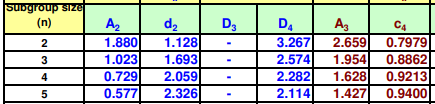# A set of 100 subgroups of measurements on productive tomato seedlings has a sum of s values of...

## Question:

A set of 100 subgroups of measurements on productive tomato seedlings has a sum of s values of 420.

If the subgroup size 6, calculate the process capability, 6?. (Answer to 3 decimal places)

## Process Capability:

The Process Capability Index is a statistical measure and it is used for the Process ability of the output within the specific limits. The value of Process Capability Index should be more for the good ability of the process.

Given that,

{eq}k = 100\\ \sum{s} = 420 n = 6 {/eq}

{eq}\bar{s} = \dfrac{\sum{s}}{k} = \dfrac{420}{100} = 4.2 {/eq}

The estimate of the process standard deviation, is defined as:

{eq}\hat{\sigma} = \dfrac{\bar{s}}{c_4} {/eq}

The value of {eq}c_4 {/eq} is calculated by the table.{eq}c_4 =0.9213 {/eq}

{eq}\hat{\sigma} = \dfrac{4.2}{0.9213} = 4.56 {/eq}

The process capability index is defined as:

{eq}C_p = \dfrac{USL-LSL}{6\hat{\sigma}} {/eq}

{eq}USL = 100+20 = 120\\ LSL = 100-20 = 80 {/eq}

So,

{eq}C_p = \dfrac{120-80}{6\times 4.56}\\ \color{blue}{C_p = 1.462} {/eq}